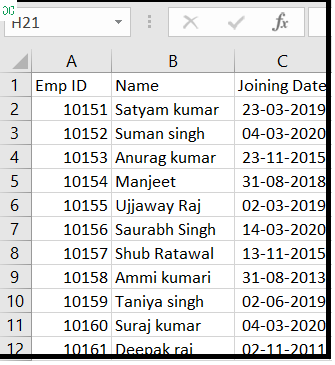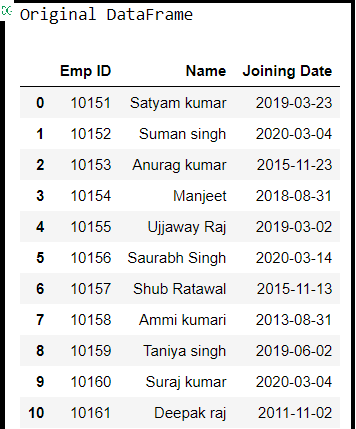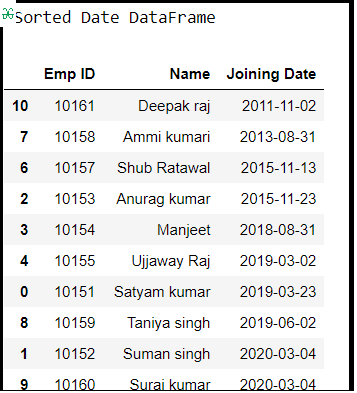Open In App

# How to sort date in excel using Pandas?

In these articles, We will discuss how to import an excel file in a single Dataframe and sort the Date in a given column on. Suppose our Excel file looks like these:sample_date.xlsx

Approach :

• Import Pandas module
• Make DataFrame from Excel file
• sort the date column with DataFrame.sort_value() function
• Display the Final DataFrame

Step 1: Importing pandas module and making DataFrame form excel.

## Python3

 `# import module``import` `pandas as pd`` ` `# making data frame from excel file``df ``=` `pd.read_excel(``'excel_work\sample_date.xlsx'``)`` ` `print``(``"Original DataFrame"``)``df`

Output :Step 2: Sorting date with DataFrame.sort_value() function.

## Python3

 `# sorting date with sort_value() function``Final_result ``=` `df.sort_values(``'Joining Date'``)`` ` `print``(``" Sorted Date DataFrame"``)``Final_result`

Output :